Request a Tool

Surface Area of a Pyramid Calculator

Choose the method by which you want to calculate the area of the pyramid and then add the values

Length
0

Formula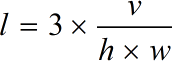• l = Length of pyramid
• v = Volume of pyramid
• w = Width of pyramid
• h = Height of pyramid

Defination / Uses

A pyramid is a solid shape with a polygonal base and triangle sides that meet at its summit. A Pyramid is a three-dimensional structure and a polyhedron.

The area, volume, base, and other parameters are automatically calculated using the applicable formula by the pyramid area calculator. It is reasonable to state that it is also a surface area of a triangular pyramid formula calculator since it composes the needed formula in run time after gathering the information.

Width
0

Formula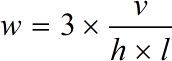• l = Length of pyramid
• v = Volume of pyramid
• w = Width of pyramid
• h = Height of pyramid

Defination / Uses

A pyramid is a solid shape with a polygonal base and triangle sides that meet at its summit. A Pyramid is a three-dimensional structure and a polyhedron.

The area, volume, base, and other parameters are automatically calculated using the applicable formula by the pyramid area calculator. It is reasonable to state that it is also a surface area of a triangular pyramid formula calculator since it composes the needed formula in run time after gathering the information.

Area
0

Formula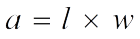• l = Length of pyramid
• a = Base Area of pyramid
• w = Width of pyramid

Defination / Uses

A pyramid is a solid shape with a polygonal base and triangle sides that meet at its summit. A Pyramid is a three-dimensional structure and a polyhedron.

The area, volume, base, and other parameters are automatically calculated using the applicable formula by the pyramid area calculator. It is reasonable to state that it is also a surface area of a triangular pyramid formula calculator since it composes the needed formula in run time after gathering the information.

Height
0

Formula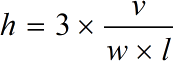• l = Length of pyramid
• v = Volume of pyramid
• w = Width of pyramid
• h = Height of pyramid

Defination / Uses

A pyramid is a solid shape with a polygonal base and triangle sides that meet at its summit. A Pyramid is a three-dimensional structure and a polyhedron.

The area, volume, base, and other parameters are automatically calculated using the applicable formula by the pyramid area calculator. It is reasonable to state that it is also a surface area of a triangular pyramid formula calculator since it composes the needed formula in run time after gathering the information.

Volume
0

Formula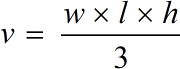• l = Length of pyramid
• v = Volume of pyramid
• w = Width of pyramid
• h = Height of pyramid

Defination / Uses

A pyramid is a solid shape with a polygonal base and triangle sides that meet at its summit. A Pyramid is a three-dimensional structure and a polyhedron.

The area, volume, base, and other parameters are automatically calculated using the applicable formula by the pyramid area calculator. It is reasonable to state that it is also a surface area of a triangular pyramid formula calculator since it composes the needed formula in run time after gathering the information.

Surface Area
0

Formula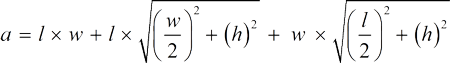• l = Length of pyramid
• a = Surface Area of pyramid
• w = Width of pyramid
• h = Height of pyramid

Defination / Uses

A pyramid is a solid shape with a polygonal base and triangle sides that meet at its summit. A Pyramid is a three-dimensional structure and a polyhedron.

The area, volume, base, and other parameters are automatically calculated using the applicable formula by the pyramid area calculator. It is reasonable to state that it is also a surface area of a triangular pyramid formula calculator since it composes the needed formula in run time after gathering the information.

Output

Lateral Surface Area
0

Formula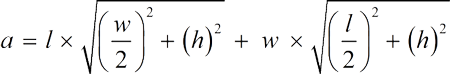• l = Length of pyramid
• a = Lateral Surface Area of pyramid
• w = Width of pyramid
• h = Height of pyramid

Defination / Uses

A pyramid is a solid shape with a polygonal base and triangle sides that meet at its summit. A Pyramid is a three-dimensional structure and a polyhedron.

The area, volume, base, and other parameters are automatically calculated using the applicable formula by the pyramid area calculator. It is reasonable to state that it is also a surface area of a triangular pyramid formula calculator since it composes the needed formula in run time after gathering the information.

How to use pyramid calcultor?

There are only two basic steps.

• First select the option from dropdown. What you need to calculate.
• Then add the other values in their corresponding fields.

Thats it! You will get your answer in just a second. use upper given formula in case of manual calculation